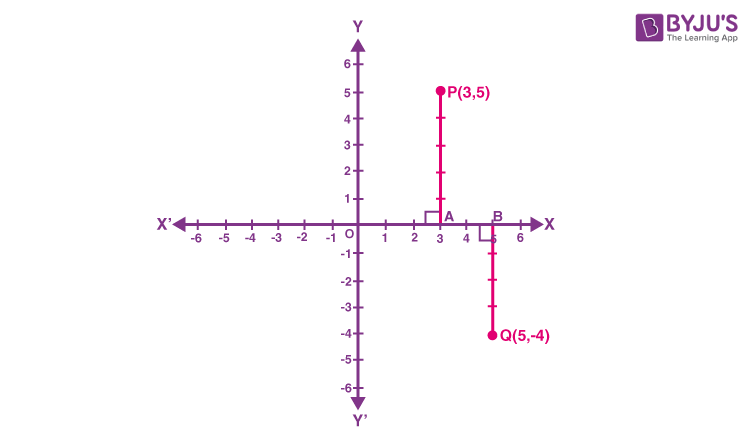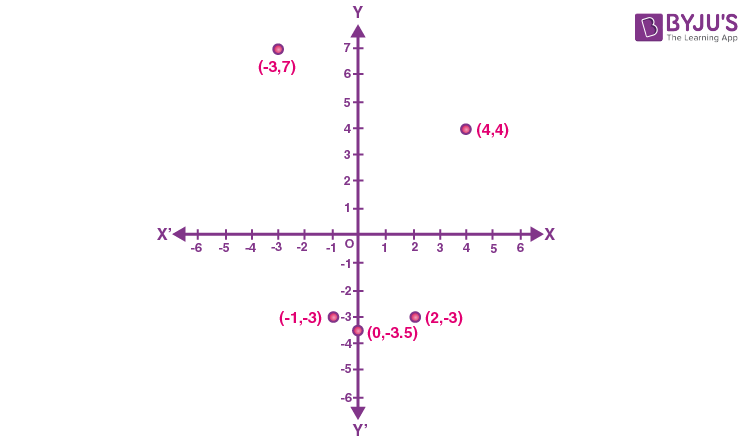# Plotting a Point in the Plane if its Coordinates are given

In Mathematics, a cartesian plane is a two-dimensional plane, which is defined by two perpendicular lines, such as horizontal line and vertical line. The cartesian plane extends infinitely in both directions. Here, we are going to learn how to plot a point in the plane if its coordinates are given with many solved examples.

## Introduction to Cartesian Plane

A cartesian plane is a two-dimensional coordinate plane defined by two perpendicular lines such as x-axis and y-axis. The horizontal number line is called x-axis and the vertical number line is called y-axis. The point of intersection of these two lines is called origin. The region of x-axis towards the right side of the origin is called positive x-axis and the region of x-axis towards the left side of the origin is called negative x-axis. Similarly, the region of y-axis above the origin is called positive y-axis and the region of y-axis below the origin is called negative y-axis. The point on the coordinate plane is represented by the ordered pair (x, y).

## Rules to Plot a Point in the Coordinate Plane

The two important rules to plot a point in the cartesian plane are given below:

1. The first coordinate in the ordered pair (x) represents the left/right movement of a point from the origin.
2. The second coordinate in the ordered pair (y) represents the up/down movement of the point from the origin.

## Solved Examples

Example 1:

Plot the coordinates (3, 5) and (5, -4) in the cartesian coordinate system.

Solution:

Step 1: Draw the coordinate axes, such as the x-axis and y-axis

Step 2: Choose the unit such that 1 centimetre represents one unit on both the x-axis and y-axis.

Step 3: The coordinate (3, 5) represents the distance from the origin to the positive x-axis is 3 units and the distance from the origin to the positive y-axis is 5 units. Now, mark these points on the coordinate plane, and name it as “P”.

Step 4: The coordinate (5, -4) represents the distance from the origin to the positive x-axis is 5 units and the distance from the origin to the negative y-axis is 4 units. Mark the coordinate point in the plane and label it as “Q”.

Step 5: Therefore, the point P (3, 5) lies in the first quadrant and the point Q (5, -4) lies in the fourth quadrant.Example 2:

Plot the following points in the cartesian plane (Use the scale: x-axis = 1 cm and y-axis = 1 cm)

 x -3 0 -1 4 2 y 7 -3.5 -3 4 -3

Solution:

From the given table, the ordered pairs formed are (-3, 7), (0, -3.5), (-1, -3), (4, 4) and (2, -3).

The point (-3, 7) lies in the 2nd quadrant

The point (0, -3.5) lies in the negative y-axis

The point (-1, -3) lies in the 3rd quadrant

The point (4, 4) lies in the 1st quadrant

The point (2, -3) lies in the 4th quadrant.

Now, plot these points on the cartesian plane.### Practice problems

1. Mark the points (5, 0), (0, 5), (2, 5), (5, 2), (–3, 5), (–3, –5), (5, –3) and (6, 1) in the cartesian coordinate plane.
2. Plot the points in the coordinate plane (– 2, 4), (3, – 1), (– 1, 0), (1, 2) and (– 3, – 5) and identify the quadrant or axis where each of the following points lies.

To learn more class-wise and Maths related concepts, register with BYJU’S – The Learning App and learn all the concepts easily by exploring more videos.

## Frequently Asked Questions on Plotting a Point in the Plane if its Coordinates are given

### What is a cartesian plane?

A cartesian plane is a coordinate plane that is defined by two axes, such as the x-axis and y-axis. The horizontal line is called the x-axis and the vertical line is called the y-axis.

### Define origin.

The point of intersection of the x-axis and y-axis is called an origin.

### What are abscissa and ordinate?

The distance of a point from the y-axis is called abscissa and the distance of a point from the x-axis is called ordinate.

### What is the coordinate of origin?

The coordinate of origin is (0, 0).

### In which quadrant is the point (2, 2) located?

A Point (2, 2) is located in the first quadrant.